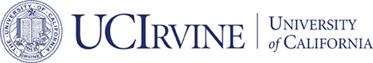# Accepted## A Finite element elasticity complex in three dimensions

### Long Chen and Xuehai Huang

#### Mathematics of Compuitation, 2022

ABSTRACT:

``` A finite element elasticity complex on tetrahedral meshes is
devised. The H1 conforming finite element is the smooth finite element
developed by Neilan for the velocity field in a discrete Stokes
complex. The symmetric div-conforming finite element is the Hu-Zhang
element for stress tensors. The construction of an H(inc)-conforming
finite element for symmetric tensors is the main focus of this
paper. The key tools of the construction are the decomposition of
polynomial tensor spaces and the characterization of the trace of the
inc operator. The polynomial elasticity complex and Koszul elasticity
complex are created to derive the decomposition of polynomial tensor
spaces. The trace of the inc operator is induced from a Green's
identity. Trace complexes and bubble complexes are also derived to
facilitate the construction. Our construction appears to be the first
H(inc)-conforming finite elements on tetrahedral meshes without
further splits.
```